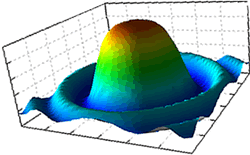Harmonics and Heterodyning of FrequenciesA perfectly symmetrical square wave produces major odd harmonics, that is, AC frequencies at 1,3,5,7,9... times its frequency. The power available in those harmonics decreases as the multiplication factor increases. For example, a 100Hz signal produces harmonics (AC frequencies) at 100, 300, 500, 700, 900, ... but at strengths of 1, 1/3, 1/5, 1/7, 1/9 ... of the original. These harmonics extend to the radio frequency range and beyond, to infinity, theoretically.

Frequencies also exhibit a heterodyning effect. This is explained by an excerpt from a web site:

----

[...] consider a sound wave of 264 cycles (middle C on the Scale of Just Intonation). In order for this tone to be perceived as both accurate and natural, one must be able to hear not just the 264 cycle tone itself, but the higher order harmonics which extend well up into the frequency range vulnerable to RF modulation.

This intermodulation creates an effect called heterodyning, where additive and subtractive tones, known as beat frequencies, are created. For example, mixing two frequencies of 100 KHz and 1 KHz results in the following frequencies being created:

100 KHz

1 KHz

101 KHz

99 KHz

These frequencies will also combine to produce beat frequencies of their own. Add in a multiplicity of RF signals and it is easy to imagine the degree to which RF alters and distorts the musical signal. The effect is especially evident in the higher frequency bands.

----

Since square waves are used, this is not just mixing two frequencies. It is mixing all the odd harmonics. Instead of producing 100 and 1, we are producing 100, 300, 500, 700, and all the additions and subtractions.

Now, combine the effects of harmonics with heterodyning with this example:

Consider a 10Hz square wave. It is producing a strong third harmonic at 30Hz (at 2/3 less power). Heterodyning means it is also producing 30 - 10 = 20Hz and 30 + 10 = 40Hz. The 5th harmonic is 50. 50-10 = 40Hz. This means that 40 gets a “boost” since it is produced in these two ways, among others. While this process goes up the scale with infinitely more numbers it produces a huge range of frequencies of varying intensities (all at less than the power at the base frequency.